Anúncio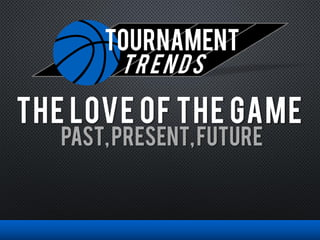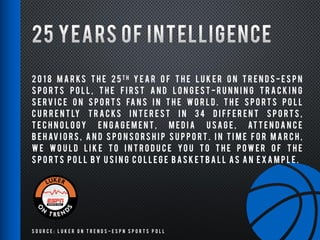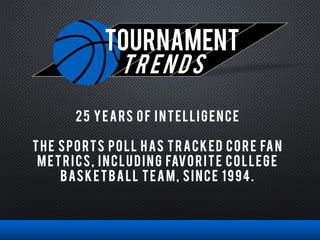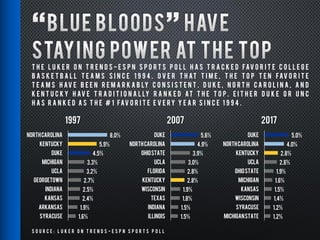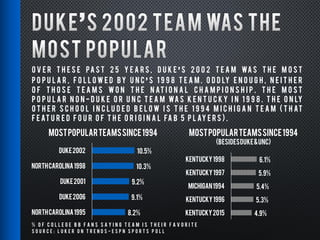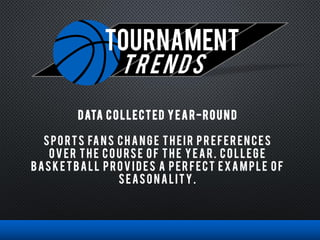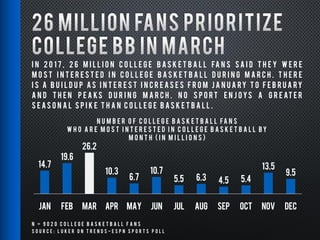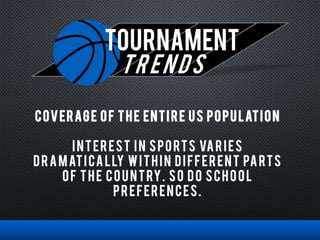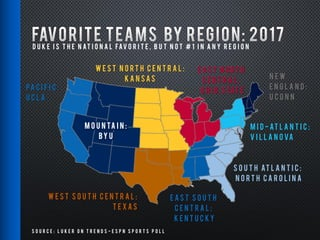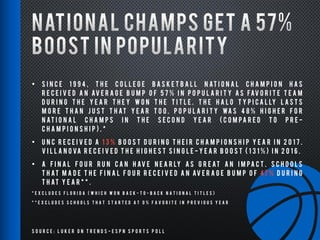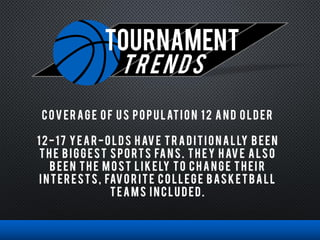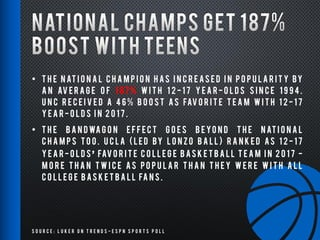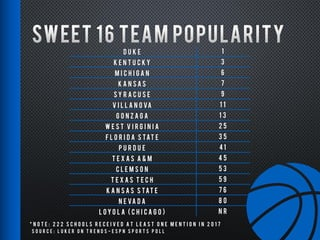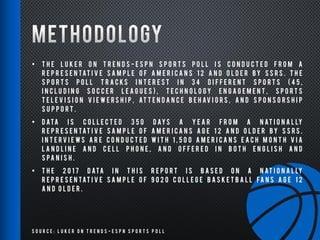1 de 16
Anúncio

### Tournament trends 2018

1. TOURNAMENT trends PAST,PRESENT,FUTURE The love of the game
2. S o u r c e : L u k e r o n T r e n d s - E S P N S p o r t s P o l l 2 0 1 8 M A R KS T H E 2 5 T H Y E A R O F T H E L U K E R O N T R E N D S - E S P N S P O RT S P O L L , T H E F I RS T A N D L O N G E S T - R U N N I N G T R A C K I N G S E RV I C E O N S P O RT S FA N S I N T H E W O R L D . T H E S P O RT S P O L L C U R R E N T LY T R A C KS I N T E R E S T I N 3 4 D I F F E R E N T S P O RT S , T E C H N O L O GY E N G A G E M E N T , M E D I A U SA G E , AT T E N D A N C E B E H AV I O RS , A N D S P O N S O RS H I P S U P P O RT . I N T I M E F O R M A R C H , W E W O U L D L I K E T O I N T R O D U C E YO U T O T H E P O W E R O F T H E S P O RT S P O L L BY U S I N G C O L L E G E B AS K E T B A L L AS A N E X A M P L E .
3. TOURNAMENT trends 25 YEARS OF INTELLIGENCE THE SPORTS POLL HAS TRACKED CORE FAN METRICS, INCLUDING FAVORITE COLLEGE BASKETBALL TEAM, SINCE 1994.
4. S o u r c e : L u k e r o n T r e n d s - E S P N S p o r t s P o l l T H E L U K E R O N T R E N D S - E S P N S P O R T S P O L L H A S T R A C K E D FAV O R I T E C O L L E G E B A S K E T B A L L T E A M S S I N C E 1 9 9 4 . O V E R T H AT T I M E , T H E T O P T E N FAV O R I T E T E A M S H AV E B E E N R E M A R K A B LY C O N S I S T E N T . D U K E , N O R T H C A R O L I N A , A N D K E N T U C K Y H AV E T R A D I T I O N A L LY R A N K E D AT T H E T O P . E I T H E R D U K E O R U N C H A S R A N K E D A S T H E # 1 FAV O R I T E E V E RY Y E A R S I N C E 1 9 9 4 . 8.0% 5.9% 4.5% 3.3% 3.2% 2.7% 2.5% 2.4% 1.9% 1.6% NorthCarolina Kentucky Duke Michigan UCLA Georgetown Indiana Kansas Arkansas Syracuse 1997 5.6% 4.9% 3.9% 3.0% 2.8% 2.8% 1.9% 1.8% 1.5% 1.5% Duke NorthCarolina OhioState UCLA Florida Kentucky Wisconsin Texas Indiana Illinois 2007 5.0% 4.0% 2.8% 2.6% 1.9% 1.6% 1.5% 1.4% 1.2% 1.2% Duke NorthCarolina Kentucky UCLA OhioState Michigan Kansas Wisconsin Syracuse MichiganState 2017
5. S o u r c e : L u k e r o n T r e n d s - E S P N S p o r t s P o l l O V E R T H E S E PA S T 2 5 Y E A R S , D U K E S 2 0 0 2 T E A M WA S T H E M O S T P O P U L A R , F O L L O W E D BY U N C S 1 9 9 8 T E A M . O D D LY E N O U G H , N E I T H E R O F T H O S E T E A M S W O N T H E N AT I O N A L C H A M P I O N S H I P . T H E M O S T P O P U L A R N O N - D U K E O R U N C T E A M WA S K E N T U C K Y I N 1 9 9 8 . T H E O N LY O T H E R S C H O O L I N C L U D E D B E L O W I S T H E 1 9 9 4 M I C H I G A N T E A M ( T H AT F E AT U R E D F O U R O F T H E O R I G I N A L FA B 5 P L AY E R S ) . 10.5% 10.3% 9.2% 9.1% 8.2% Duke2002 NorthCarolina1998 Duke2001 Duke2006 NorthCarolina1995 MOSTPOPULARTEAMSSINCE1994 6.1% 5.9% 5.4% 5.3% 4.9% Kentucky1998 Kentucky1997 Michigan1994 Kentucky1996 Kentucky2015 MostPopularTeamsSince1994 (BesidesDuke&UNC) % o f C o l l e g e B B F a n s s a y i n g t e a m i s t h e i r f a v o r i t e
6. TOURNAMENT trends DATA COLLECTED YEAR-ROUND SPO RTS FANS CHANG E TH EI R PR EFER ENCES OVER TH E COURSE OF TH E YEAR. COLLEGE BASKETBALL PROVI DES A PER FECT EXAM PLE O F SEASO NALITY.
7. S o u r c e : L u k e r o n T r e n d s - E S P N S p o r t s P o l l 14.7 19.6 26.2 10.3 6.7 10.7 5.5 6.3 4.5 5.4 13.5 9.5 JAN FEB MAR APR MAY JUN JUL AUG SEP OCT NOV DEC N U M B E R O F C o l l e g e B A S K E T B A L L fa n s w h o a r e m o s t i n t e r e s t e d i n C o l l e g e B A S K E T B A L L b y m o n t h ( i n m i l l i o n s ) N = 9 0 2 0 C o l l e g e B a s k e t b a l l F a n s I n 2 0 1 7 , 2 6 m i l l i o n C o l l e g e B a s k e t b a l l fa n s s a i d t h e y w e r e m o s t i n t e r e s t e d i n C o l l e g e B a s k e t b a l l d u r i n g M a r c h . T h e r e i s a b u i l d u p a s i n t e r e s t i n c r e a s e s f r o m J a n u a ry t o F e b r u a ry a n d t h e n p e a ks d u r i n g M a r c h . N o s p o r t e n j o ys a g r e at e r s e a s o n a l s p i k e t h a n C o l l e g e B a s k e t b a l l .
8. TOURNAMENT trends COVERAGE OF THE ENTIRE US POPULATION INTEREST IN SPORTS VARIES DRAMATICALLY WITHIN DIFFERENT PARTS OF THE COUNTRY. SO DO SCHOOL PREFERENCES.
9. S o u r c e : L u k e r o n T r e n d s - E S P N S p o r t s P o l l D u k e i s t h e n at i o n a l fav o r i t e , b u t n o t # 1 i n a n y r e g i o n Pa c i f i c : U C L A N e w E n g l a n d : U C o n n M i d - At l a n t i c : V i l l a n o va E a s t N o r t h C e n t r a l : O h i o S tat e W e s t N o r t h C e n t r a l : K a n s a s S o u t h At l a n t i c : N o r t h C a r o l i n a E a s t S o u t h C e n t r a l : K e n t u c k y W e s t S o u t h C e n t r a l : T e x a s M o u n ta i n : BY U
10. TOURNAMENT trends INTELLIGENCE BEYOND DATA EVERY MONTH LUKER ON TRENDS PROVIDES INTELLIGENCE THAT LOOKS AT TRENDS AND EMERGING ISSUES IN GREATER DEPTH.
11. S o u r c e : L u k e r o n T r e n d s - E S P N S p o r t s P o l l • S I N C E 1 9 9 4 , T H E C O L L E G E B A S K E T B A L L N AT I O N A L C H A M P I O N H A S R E C E I V E D A N AV E R A G E B U M P O F 5 7 % I N P O P U L A R I T Y A S FAV O R I T E T E A M D U R I N G T H E Y E A R T H E Y W O N T H E T I T L E . T H E H A L O T Y P I C A L LY L A S T S M O R E T H A N J U S T T H AT Y E A R T O O . P O P U L A R I T Y WA S 4 8 % H I G H E R F O R N AT I O N A L C H A M P S I N T H E S E C O N D Y E A R ( C O M PA R E D T O P R E - C H A M P I O N S H I P ) . * • U N C R E C E I V E D A 1 3 % B O O S T D U R I N G T H E I R C H A M P I O N S H I P Y E A R I N 2 0 1 7 . V I L L A N O VA R E C E I V E D T H E H I G H E S T S I N G L E - Y E A R B O O S T ( 1 3 1 % ) I N 2 0 1 6 . • A F I N A L F O U R R U N C A N H AV E N E A R LY A S G R E AT A N I M PA C T . S C H O O L S T H AT M A D E T H E F I N A L F O U R R E C E I V E D A N AV E R A G E B U M P O F 47 % D U R I N G T H AT Y E A R * * . * E X C L U D E S F L O R I D A ( W H I C H W O N B A C K - T O - B A C K N A T I O N A L T I T L E S ) * * E X C L U D E S S C H O O L S T H A T S T A R T E D A T 0 % F A V O R I T E I N P R E V I O U S Y E A R
12. TOURNAMENT trends COVERAG E O F US PO PU LATIO N 12 AN D O LDER 12-17 YEAR-O LDS HAVE TRADITIO NALLY BEEN TH E BIGGEST SPORTS FANS. TH EY HAVE ALSO BEEN TH E MOST LI KELY TO CHANG E TH EI R I NTER ESTS, FAVO R ITE CO LLEGE BASKETBALL TEAMS I NCLU DED.
13. S o u r c e : L u k e r o n T r e n d s - E S P N S p o r t s P o l l • T H E N AT I O N A L C H A M P I O N H AS I N C R E AS E D I N P O P U L A R I T Y BY A N AV E R A G E O F 1 8 7 % W I T H 1 2 - 1 7 Y E A R - O L D S S I N C E 1 9 9 4 . U N C R E C E I V E D A 4 6 % B O O S T AS FAV O R I T E T E A M W I T H 1 2 - 1 7 Y E A R - O L D S I N 2 0 1 7 . • T H E B A N D WA G O N E F F E C T G O E S B E YO N D T H E N AT I O N A L C H A M P S T O O . U C L A ( L E D BY L O N Z O B A L L ) R A N K E D AS 1 2 - 1 7 Y E A R - O L D S FAV O R I T E C O L L E G E B AS K E T B A L L T E A M I N 2 0 1 7 - M O R E T H A N T W I C E AS P O P U L A R T H A N T H E Y W E R E W I T H A L L C O L L E G E B AS K E T B A L L FA N S .
14. S o u r c e : L u k e r o n T r e n d s - E S P N S p o r t s P o l l D u k e 1 K e n t u c k y 3 M i c h i g a n 6 K a n s a s 7 S y r a c u s e 9 V i l l a n o va 1 1 G o n z a g a 1 3 W e s t V i r g i n i a 2 5 F l o r i d a S tat e 3 5 P u r d u e 41 T e x a s A & M 4 5 C l e m s o n 5 3 T e x a s T e c h 5 9 K a n s a s S tat e 7 6 N e va d a 8 0 L o y o l a ( C h i c a g o ) N R * N o t e : 2 2 2 s c h o o l s r e c e i v e d a t l e a s t o n e m e n t i o n i n 2 0 1 7
15. S o u r c e : L u k e r o n T r e n d s - E S P N S p o r t s P o l l • T H E L U K E R O N T R E N D S - E S P N S P O R T S P O L L I S C O N D U C T E D F R O M A R E P R E S E N TAT I V E S A M P L E O F A M E R I C A N S 1 2 A N D O L D E R B Y S S R S . T H E S P O R T S P O L L T R A C K S I N T E R E S T I N 3 4 D I F F E R E N T S P O R T S ( 4 5 , I N C L U D I N G S O C C E R L E A G U E S ) , T E C H N O L O G Y E N G A G E M E N T , S P O R T S T E L E V I S I O N V I E W E R S H I P , AT T E N D A N C E B E H AV I O R S , A N D S P O N S O R S H I P S U P P O R T . • D ATA I S C O L L E C T E D 3 5 0 D AY S A Y E A R F R O M A N AT I O N A L LY R E P R E S E N TAT I V E S A M P L E O F A M E R I C A N S A G E 1 2 A N D O L D E R B Y S S R S . I N T E R V I E W S A R E C O N D U C T E D W I T H 1 , 5 0 0 A M E R I C A N S E A C H M O N T H V I A L A N D L I N E A N D C E L L P H O N E , A N D O F F E R E D I N B O T H E N G L I S H A N D S PA N I S H . • T H E 2 0 1 7 D ATA I N T H I S R E P O R T I S B A S E D O N A N AT I O N A L LY R E P R E S E N TAT I V E S A M P L E O F 9 0 2 0 C O L L E G E B A S K E T B A L L FA N S A G E 1 2 A N D O L D E R .
16. S o u r c e : L u k e r o n T r e n d s - E S P N S p o r t s P o l l MELISSA HERRMANN P R E S I D E N T M H E R R M A N N @ S S RS . C O M @ H E R R M A N N M J CHAD MENEFEE S E N I O R PA RT N E R C H A D @ L U K E R O N T R E N D S . C O M
Anúncio